Equation of motion for a particle: Newton’s 2nd law

Newton’s 2nd law: The resultant of all the forces acting on a particle is proportional to the acceleration of the particle.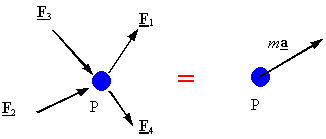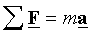Newton’s 3rd law: Each action has a reaction equal in magnitude, but opposite in direction

Equations of motion in a rectangular coordinate system: In a rectangular coordinate system the equations of motion can be represented by three scalar equations:Equations of motion in normal and tangential (natural) coordinate systems: In a natural coordinate system the equations of motion can be represented by three scalar equations: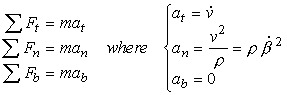Equations of motion in Cylindrical coordinate systems: In a cylindrical coordinate system the equations of motion can be represented by three scalar equations: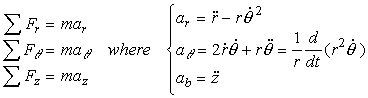Free-Body diagram: A diagram showing the particle under consideration and all the forces acting on the  particle. Each force in this diagram must be labeled.

Gravity: The force of gravity is equal to the mass times the acceleration of gravity and is applied at the center of gravity (CG) of the body.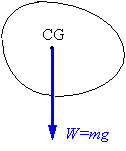String or cable: A mechanical device that can only transmit a tensile force along itself.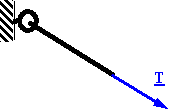Linear spring: A mechanical device which exerts a force proportional to its extension along its line of action.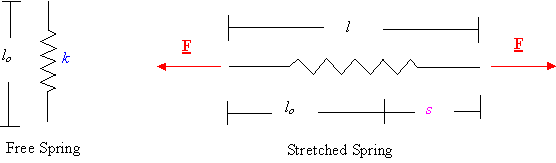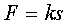Frictionless and massless pulleys: For a frictionless and massless  pulley, the tension in the cable is the same on both sides of the pulley.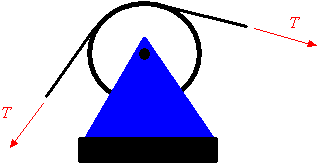Copy and distribute freely for personal use only

Department of Engineering Mechanics, University of Nebraska, Lincoln, NE 68588-0526